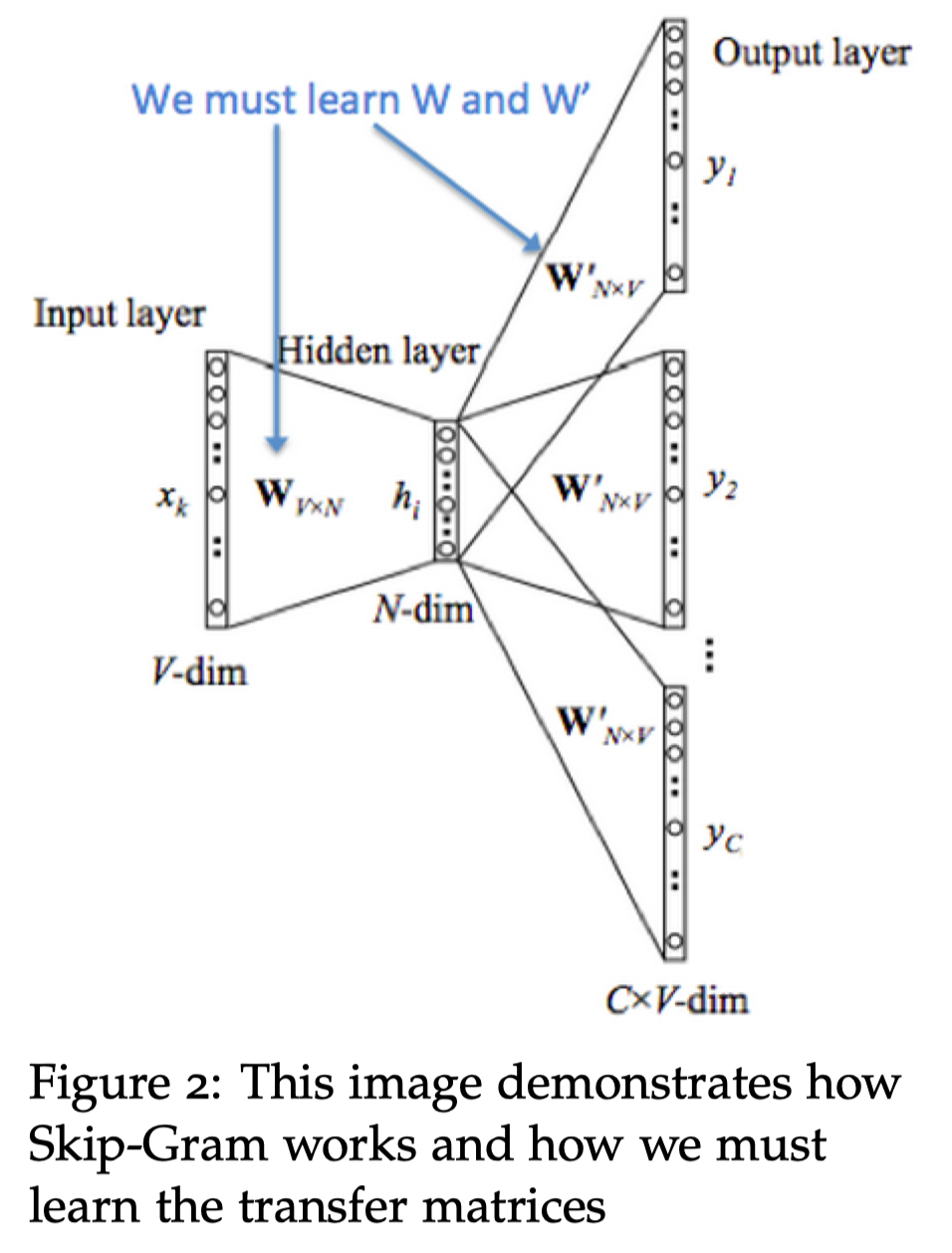# 1 Introduction of Natural Language Processing

Natural Language is a discrete/symbolic/categorical system.

There are many different levels in NLP. For instance:

Easy:

• Spell Checking
• Keywords Search

• Finding Synonyms

• Synonym: 同义词 noun

Medium

• Parsing information from websites, documents, etc.

• Parse: 对句子进行语法分析 verb

Hard

• Machine Translation
• Semantic Analysis (What is the meaning of query statement?)
• Coreference (e.g. What does “he” or “it” refer to given a document?)

Coreference: 共指关系，指代的词

## 1.2 How to represent words?

### 1.2.1 WordNet

• Use e.g. WordNet, a thesaurus containing lists of synonym sets and hypernyms (“is a” relationships).

hypernym: 上位词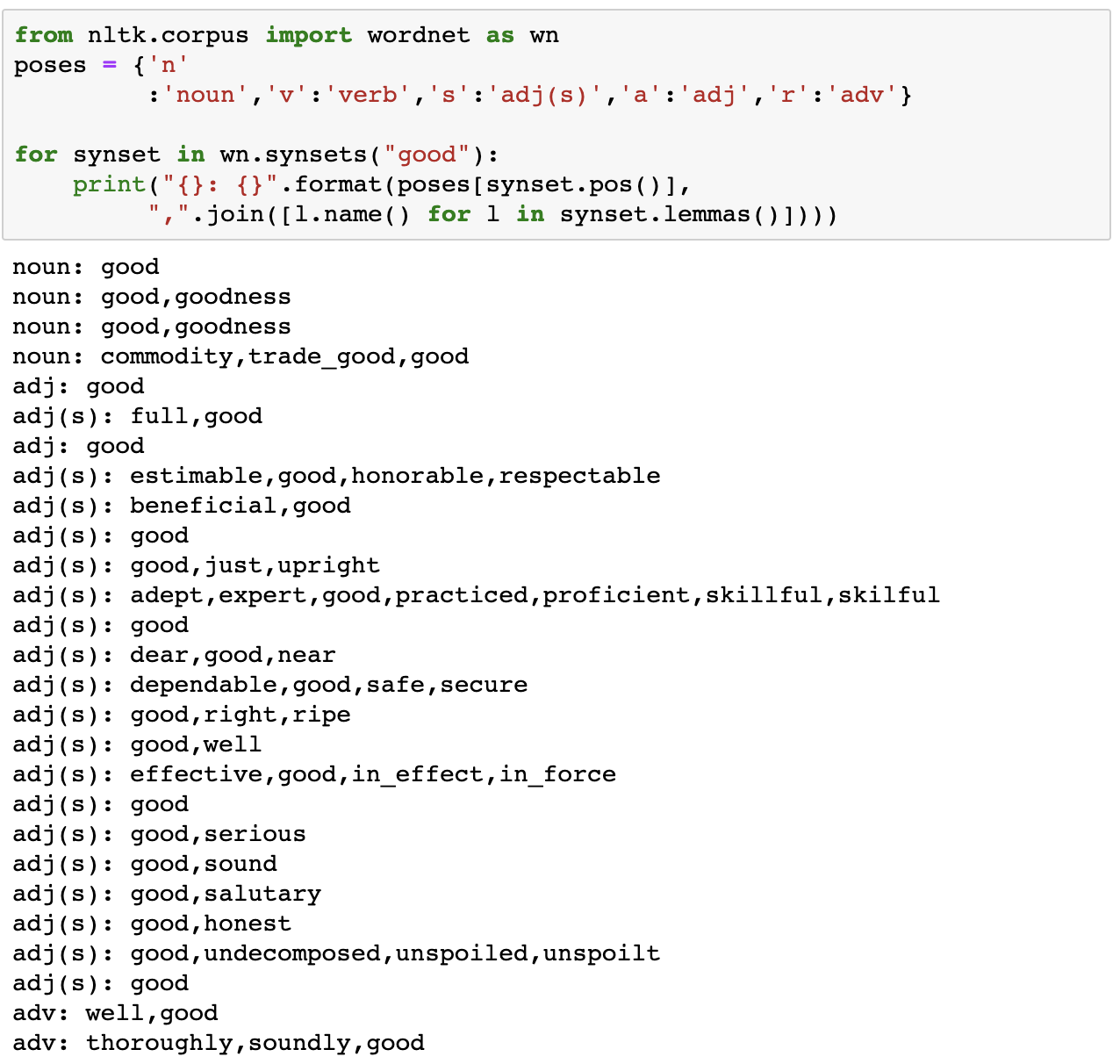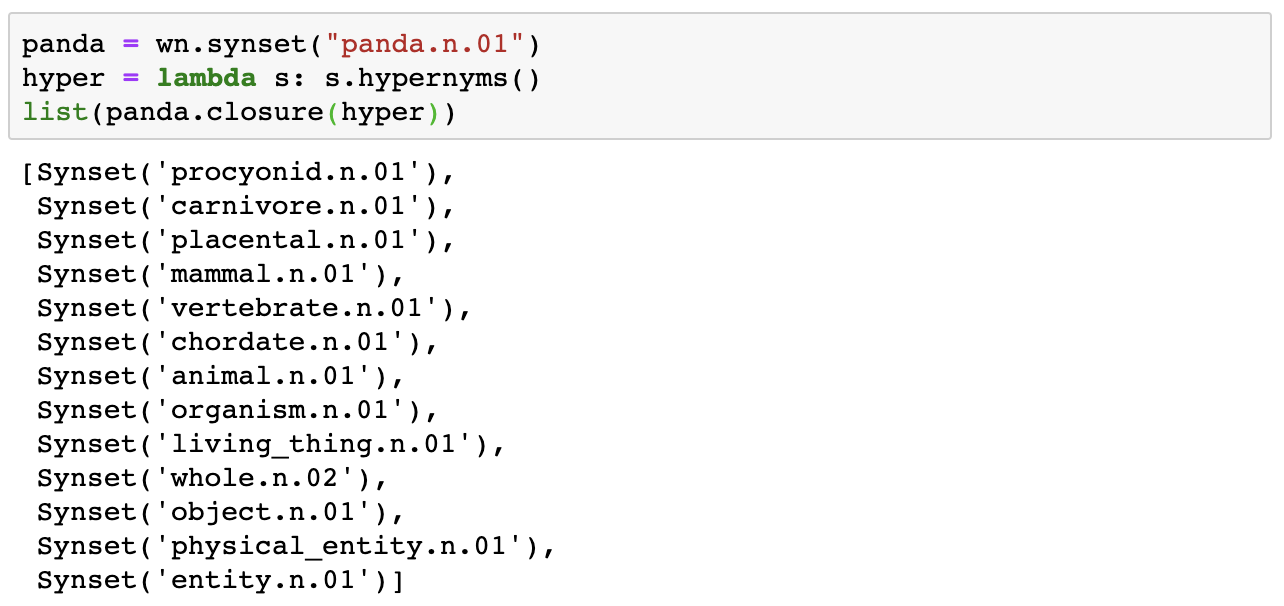Problems with resources like WordNet

• Great as a resource but missing nuance (e.g. “proficient” is listed as a synonym for “good”, This is only correct in some contexts.)
• Missing new meanings of words
• Subjective
• Requires human labor to create and adapt
• Can’t compute accurate word similarity

### 1.2.2 Representing words as discrete symbols

In traditional NLP, we regard words as discrete symbols: hotel, conference, motel – a localist representation.

Words can be represented by one-hot vectors, one-hot means one 1 and the rest are 0. Vector dimension = number of words in vocabulary.

• Problem with words as discrete symbols:

For example, if user searches for “Seattle motel”, we would like to match documents containing “Seattle hotel”.

But:

These two vectors are orthogonal. But for one-hot vectors didn’t shown there’s natural notion of similarity.

• Solution:

• Could try to rely on WordNet’s list of synonyms to get similarity?
• But it is well-known to fail badly: incompleteness, etc.
• Instead: Learn to encode similarity in the vectors themselves

### 1.2.3 Representing words by their context

• Distributional semantics: A word’s meaning is given by the words that frequently appear close-by.
• “You shall know a word by the company it keeps”(J.R. Firth 1957: 11)
• One of the most successful ideas of modern statistical NLP
• When a word “w” appears in a text, its context is the set of words that appear nearby(within a fixed-size windows).
• Use the many contexts of “w” to build up a representation of “w“.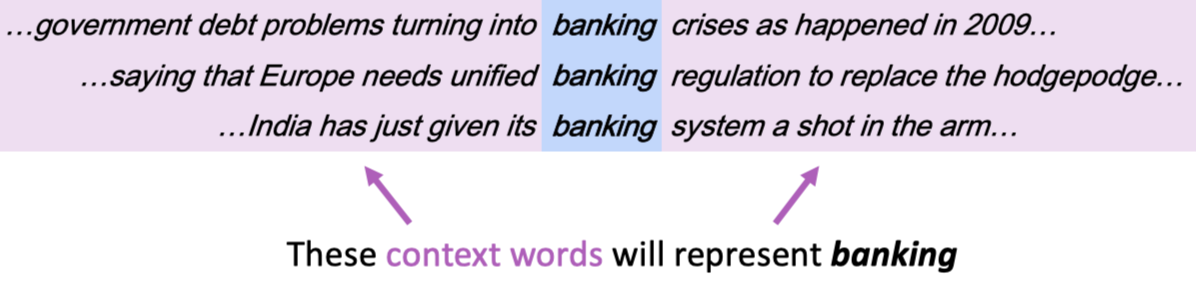# 2 Word2Vec

• Word Vectors: In word2vec, a distributed representation of a word is used. Each word is represented by a distribution of weights across those elements. So instead of a one-to-one mapping between an element in the vector and a word, the representation of a word is spread across all of the elements in the vector, and each element in the vector contributes to the definition of many words.

Note: Word vectors are sometimes called word embeddings or word representations. They are a distributed representation.

## 2.1 Word2Vec: Overview

Word2vec (Mikolov et al. 2013) is a framework for learning word vectors.

Idea:

• We have a large corpus of text
• Every word in a fixed vocabulary is represented by a vector
• Go through each position t in the text, which has a center word c and context (“outside”) words o
• Use the similarity of the word vectors for c and o to calculate the probability of o given c (or vice versa)
• Keep adjusting the word vectors to maximize this probability

## 2.2 Word2Vec: Objective Function

Example windows and process for computing:

P(w_{t+j}|w_t)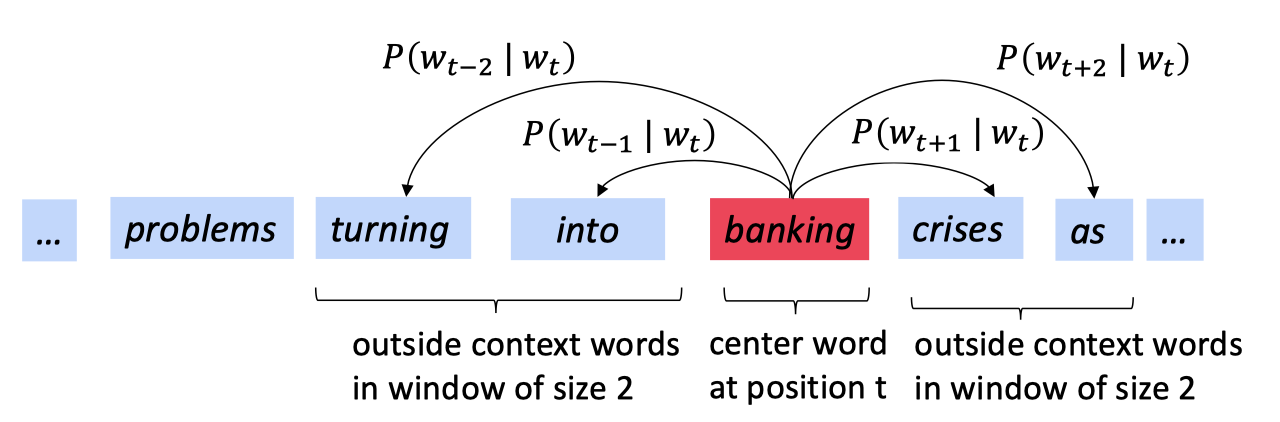For each position, t=1, \dots , T , predict context words within a window of fixed size m, given center word w_j.

Likelihood=L(\theta)=\prod_{t=1}^T\prod_{-m\leq j \leq m}P(w_{t+j}|w_t;\theta)

where \theta is all variables to be optimized.

• The objective function J(\theta) is the average negative log likelihood:

Sometimes, objective function called cost or loss function.

J(\theta)=-\frac{1}{T}\log L(\theta)=-\frac{1}{T}\sum_{t=1}^{T}\sum_{-m\leq j \leq m}\log P(w_{t+j}|w_t;\theta)

So, our target that maximizing predictive accuracy is equivalent to minimizing objective function.

Minimizing objective function \Leftrightarrow Maximizing predictive accuracy.

How to calculate P(w_{t+j}|w_t;\theta) ?

To calculate P(w_{t+j}|w_t;\theta) use two vectors per word w:

• v_w when w is a center word
• u_w when w is a context word

Then for a center word c and a context word o:

P(o|c)=\frac{\exp(u_o^Tv_c)}{\sum_{w\in V}\exp(u_w^Tv_c)}

In the formula above Exponentiation makes anything positive, u_o^Tv_c is dot product that compares similarity of o and c. Large dot product = Large probability. Denominator \sum_{w\in V}\exp(u_w^Tv_c)normalize over entire vocabulary to give probability distribution.

The follow is an example of softmax function \mathbb{R}\rightarrow\mathbb{R}

softmax(x_i)=\frac{\exp(x_i)}{\sum_{j=1}^{n}\exp(x_j)}=p_i

Softmax Function apply the standard exponential function to each element x_i of the input vector x and normalize these values by dividing by the sum of all these exponentials; this normalization ensures that the sum of the components of the output vector p is 1.

The softmax function above maps arbitrary values x_i to a probability distribution p_i

• max because it amplifies probability of largest x_i
• soft because it still assigns some probability to smaller x_i
• Frequently used in Deep Learning.

\theta represents all model parameters. For example we have V words and each vector of word has d dimensional. And every word such as word w have two vectors, one is the center word vector v_w and another is the context vector u_w. So, \theta \in \mathbb{R}^{2dV} , we can optimize these parameters by walking down the gradient.

\frac{\partial x^Ta}{\partial x}=\frac{\partial a^Tx}{\partial x}=a

Write out with indices can proof it.

We need to minimize

J(\theta)=-\frac{1}{T}\log L(\theta)=-\frac{1}{T}\sum_{t=1}^{T}\sum_{-m\leq j \leq m, j\neq0}\log P(w_{t+j}|w_t;\theta)

minimize J(\theta) is equivalent to minimize \log P(o|c)=\log \frac{\exp(u_o^Tv_c)}{\sum_{w=1}^V\exp(u_w^Tv_c)}.

Take the partial of \log P(o|c)=\log \frac{\exp(u_o^Tv_c)}{\sum_{w=1}^V\exp(u_w^Tv_c)}, we can get the follow results:

\log P(o|c)=\log \frac{\exp(u_o^Tv_c)}{\sum_{w=1}^V\exp(u_w^Tv_c)}=\log \exp(u_o^Tv_c)-\log \sum_{w=1}^V\exp(u_w^Tv_c)

\frac{\partial P(o|c)}{\partial v_c}=u_o-\frac{\sum_{x=1}^V u_x\exp(u_w^Tv_c)}{\sum_{w=1}^V\exp(u_w^Tv_c)}=u_o-\sum_{x=1}^Vu_xP(x|c)

The statement above illustrate the skip-gram language model and how to update the parameters of J(\theta).